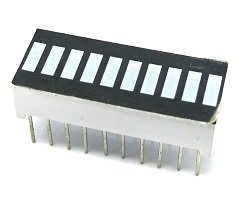# Bar Graph

## Introduction

This project explores a couple of ways of simulating and using a bar graph with the Arduino. Bar graph components like the following one can be used,This type of bar graph is basically just 10 LEDs in a row with anodes on one side of the component and cathodes on the other.

The same thing can be created by just placing 10 LEDs on a breadboard. If you have LEDs smaller than 5mm, you may be able to get them closer together on the breadboard. If not, just space out as you see in the diagrams.

## You Will Need

• 10 x LEDs or 1 x 10 Segement Bar Graph
• 10 x 330 Ohm Resistors
• 10K Trimmer Potentiometer
• 1 x Piezo Buzzer

## Making The Circuit – Bar Graph

The first job is to connect up all of those LEDs and resistors to make the bar graph.## Programming The Arduino – Bar Graph

Here is some code to test that the bar graph has been wired correctly.

```int ledPin[] = {1,2,3,4,5,6,7,8,9,10}; void setup() { for (int i =0;i<10;i++) { pinMode(ledPin[i], OUTPUT); } }```

``` void loop() { upanddown(); delay(1000); oneatatime(); delay(1000); } void alloff() { for (int i =0;i<10;i++) { digitalWrite(ledPin[i], LOW); } } void allon() { for (int i =0;i<10;i++) { digitalWrite(ledPin[i], HIGH); } } void showUpTo(byte numtoshow) { alloff(); for (byte i = 0;i<numtoshow;i++) { digitalWrite(ledPin[i],HIGH); } ```

```} void upanddown() { for (int i =0;i<10;i++) { digitalWrite(ledPin[i], HIGH); delay(100); } for (int i=9;i>=0;i--) { digitalWrite(ledPin[i], LOW); delay(100); } } void oneatatime() { for (int i =0;i<10;i++) { digitalWrite(ledPin[i], HIGH); delay(100); digitalWrite(ledPin[i], LOW); delay(100); } } ```

## Making The Circuit – Trimpot

Adding a trimpot to control the bar graph allows the bar graph to be used as an indicator for the position of the potentiometer.

For more detail: Bar Graph